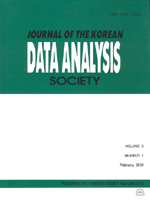상세검색
최근 검색어 전체 삭제
다국어입력
즐겨찾기0KCI등재 학술저널

# Variance Function Estimation using SVM and Jack-Knife Method

• 등재여부 : KCI등재
• 2011.10
• 2243 - 2251 (9 pages)

We propose an estimation method of variance functions in the heteroscedastic regression. To obtain more stable variance function estimation we use the averages of squared jack-knifed residuals obtained from support vector machine. We use the iteratively reweighted least squares procedure to estimate the regression function in support vector machine for easy model selection method which employs the generalized approximate cross validation techniques for choosing the hyper-parameters which affect the performance of the regression method. An artificial example and a real example are provided to indicate the usefulness of the proposed method for the estimation of variance functions.

1. Introduction

2. Support Vector Machine

3. Variance function estimation by Jack-knife method

4. Numerical Studies

5. Conclusions

References